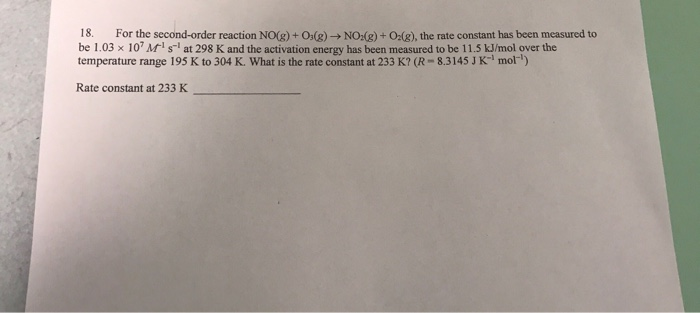# Question & Answer: For the second order reaction NO(g) + O_3(g) rightarrow NO_2(g) + O_2(g), the rate…..Please answer and explain! Thank you!

For the second order reaction NO(g) + O_3(g) rightarrow NO_2(g) + O_2(g), the rate constant has been measured to be 1.03 times 10^7 M^-1 s^-1 at 298 K and the activation energy has been measured to be 11.5 kJ/mol over the temperature range 195 K to 304 K. What is the rate constant at 233 K? (R = 8.3145 J K^-1 mol^-1) Rate constant at 233 K _____

We have second order reaction NO(g) + O3(g) → NO2(g) + O2(g)
Rate constant k1 = 1.03 x 107 M-1 s-1 at T1 = 298 K
Activation energy Ea= 11.5 KJ/mol = 11500 J/mol
Universal gas constant R=8.314 JK-1 mol-1
T2 = 233 K and we need to find out rate constant k2 =?

The Arrhenius equation in the form of two different temperatures is:

Don't use plagiarized sources. Get Your Custom Essay on
Question & Answer: For the second order reaction NO(g) + O_3(g) rightarrow NO_2(g) + O_2(g), the rate…..
GET AN ESSAY WRITTEN FOR YOU FROM AS LOW AS \$13/PAGE

ln(k2/k1) = – Ea/R ((1/T2) – (1/T1))

ln (k2/1.03 x 107 M-1 s-1) = -(11500 J/mol)/(8.314 JK-1 mol-1){(1/233) – (1/298)}
ln (k2/1.03 x 107 M-1 s-1)= -1.295
k2 = 2.82 x 106 M-1 s-1
Therefore,, the rate constant at 233K = 2.82 x 106 M-1 s-1